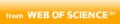Autor(en)
Wang, Wei; Xu, Xuejun
Titel
On the convergence of a two-level preconditioned Jacobi--Davidson method for eigenvalue problems
Teil von
• Mathematics of computation, 2019-09-01, Vol.88 (319), p.2295-2324
Ort / Verlag
PROVIDENCE: AMER MATHEMATICAL SOC
Quelle
Web of ScienceBeschreibungen
In this paper, we shall give a rigorous theoretical analysis of the two-level preconditioned Jacobi-Davidson method for solving the large scale discrete elliptic eigenvalue problems, which was essentially proposed by Zhao, Hwang, and Cai in 2016. Focusing on eliminating the error components in the orthogonal complement space of the target eigenspace, we find that the method could be extended to the case of the 2mth order elliptic operator ( m=1,2). By choosing a suitable coarse space, we prove that the method holds a good scalability and we obtain the error reduction \gamma =c(1-C\frac {\delta ^{2m-1}}{H^{2m-1}}) in each iteration, where C is a constant independent of the mesh size h and the diameter of subdomains H , \delta is the overlapping size among the subdomains, and c\rightarrow 1 decreasingly as H\rightarrow 0 . Moreover, the method does not need any assumption between H and h . Numerical results supporting our theory are given.
Format
Sprache(n)
Englisch
Identifikator(en)
ISSN: 0025-5718
ISSN: 1088-6842
DOI: 10.1090/mcom/3403